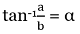# valarray atan() Function in C++ with Examples

C++ valarray atan() Function: Here, we will learn about the atan() function, its usages, syntax and examples.
Submitted by Shivang Yadav, on May 20, 2022

The valarray class in C++ is a special container that is used for holding elements like an array and performing operations on them.

Mathematical Arc Tangent

Mathematically, arc tangent is the inverse of cosine function. The arc tangent often known as tan inverse is used to evaluate the value of angle using the given ratio of side opposite to the angle and side adjacent to the angle.

Arc tangent is denoted as tan-1 and formulated as,Where,

• a is the length of side opposite to the angle
• b is the length of side adjacent to the angle
• α is the angle

## std::atan(std::valarray) Function

The atan() function in the valarray class is a function used for creating a valarray consisting of elements calculated as tan-1 for each element of the original valarray.

Syntax:

```template< class T >
valarray<T> atan( const valarray<T>& va );
```

Parameter(s): It takes one parameter the valarray on which the mathematical operation is to be applied.

Return Value: It returns a valarray consisting of tan-1 value of each element of the initial valarray.

This is all the basics about the valarray function. Moving ahead, we are now creating programs to test value on the atan() function and results on different sets of values.

## C++ valarray atan() Function Example 1:

```#include <iostream>
#include <valarray>
using namespace std;

int main()
{
// Declaring valarray
valarray<int> myvalarr = { 16, 314, 0, 1, 2 };

// Printing the elements of valarray
cout << "The elements of orignal valarray are : ";
for (int& ele : myvalarr)
cout << ele << " ";

valarray<int> atanvalarray = atan(myvalarr);
cout << "\nThe elements of atan valarray are : ";
for (int& ele : atanvalarray)
cout << ele << " ";

return 0;
}
```

Output:

```The elements of orignal valarray are : 16 314 0 1 2
The elements of atan valarray are : 1 1 0 0 1
```

## C++ valarray atan() Function Example 2:

```#include <iostream>
#include <valarray>
using namespace std;

int main()
{
// Declaring valarray
valarray<double> myvalarr = { 1.6, -0.5, 0, 1, -1 };

// Printing the elements of valarray
cout << "The elements of orignal valarray are : ";
for (double& ele : myvalarr)
cout << ele << " ";

valarray<double> atanvalarray = atan(myvalarr);
cout << "\nThe elements of atan valarray are : ";
for (double& ele : atanvalarray)
cout << ele << " ";

return 0;
}
```

Output:

```The elements of orignal valarray are : 1.6 -0.5 0 1 -1
The elements of atan valarray are : 1.0122 -0.463648 0 0.785398 -0.785398
```

Preparation

What's New

Top Interview Coding Problems/Challenges!